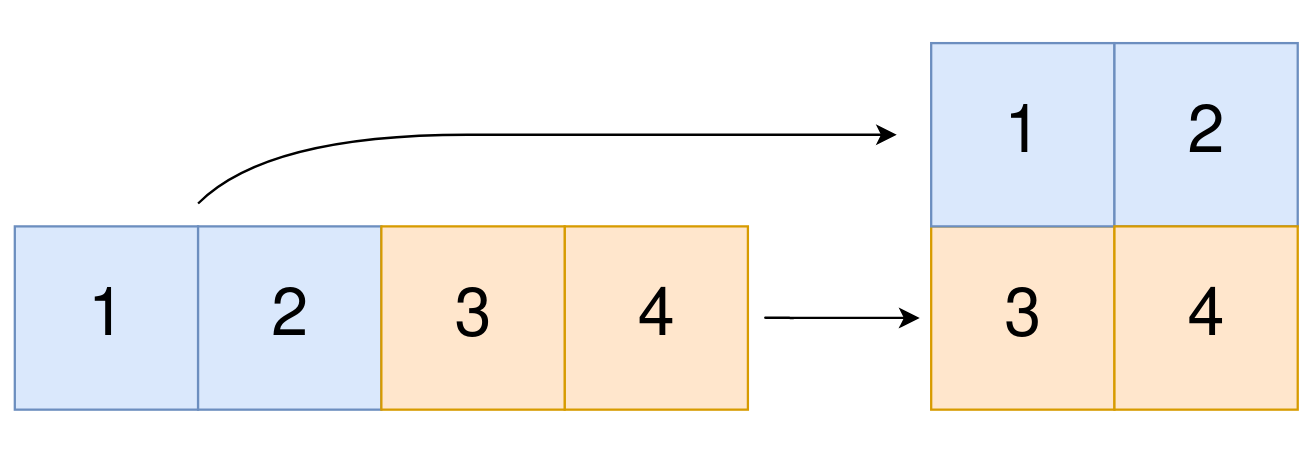2022. Convert 1D Array Into 2D Array

#### QUESTION:

You are given a 0-indexed 1-dimensional (1D) integer array original, and two integers, m and n. You are tasked with creating a 2-dimensional (2D) array with m rows and n columns using all the elements from original.

The elements from indices 0 to n - 1 (inclusive) of original should form the first row of the constructed 2D array, the elements from indices n to 2 * n - 1 (inclusive) should form the second row of the constructed 2D array, and so on.

Return an m x n 2D array constructed according to the above procedure, or an empty 2D array if it is impossible.

Example 1:``````Input: original = [1,2,3,4], m = 2, n = 2
Output: [[1,2],[3,4]]
Explanation: The constructed 2D array should contain 2 rows and 2 columns.
The first group of n=2 elements in original, [1,2], becomes the first row in the constructed 2D array.
The second group of n=2 elements in original, [3,4], becomes the second row in the constructed 2D array.
``````

Example 2:

``````Input: original = [1,2,3], m = 1, n = 3
Output: [[1,2,3]]
Explanation: The constructed 2D array should contain 1 row and 3 columns.
Put all three elements in original into the first row of the constructed 2D array.
``````

Example 3:

``````Input: original = [1,2], m = 1, n = 1
Output: []
Explanation: There are 2 elements in original.
It is impossible to fit 2 elements in a 1x1 2D array, so return an empty 2D array.
``````

Constraints:

``````1 <= original.length <= 5 * 104
1 <= original[i] <= 105
1 <= m, n <= 4 * 104
``````

#### SOLUTION:

``````class Solution {
func construct2DArray(_ original: [Int], _ m: Int, _ n: Int) -> [[Int]] {
if (m * n != original.count) {
return []
}
var result:[[Int]] = []
var index = 0
for _ in 0..<m {
var tmpArr:[Int] = []
for _ in 0..<n {
tmpArr.append(original[index])
index += 1
}
result.append(tmpArr)
}
return result
}
}
``````